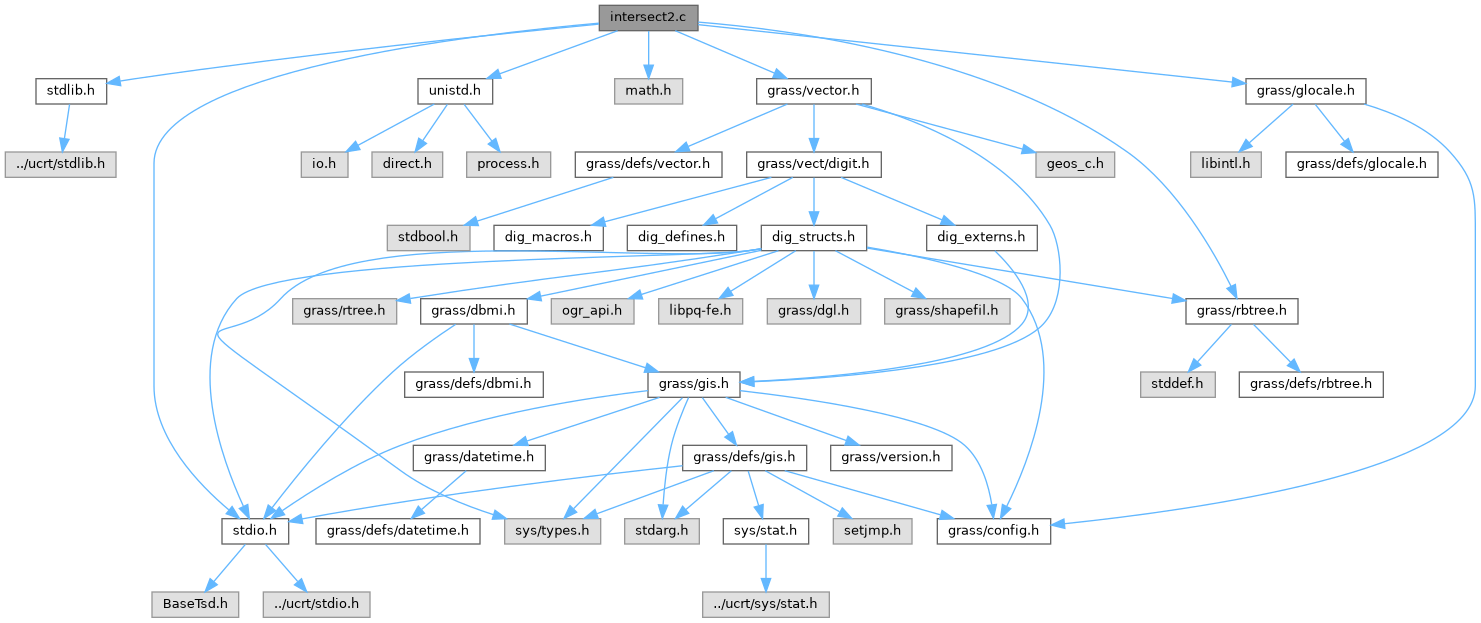GRASS GIS 8 Programmer's Manual  8.2.2dev(2023)-5c00b4e944
intersect2.c File Reference

Vector library - intersection. More...

`#include <stdlib.h>`
`#include <stdio.h>`
`#include <unistd.h>`
`#include <math.h>`
`#include <grass/vector.h>`
`#include <grass/rbtree.h>`
`#include <grass/glocale.h>`
Include dependency graph for intersect2.c:Go to the source code of this file.

## Macros

#define QEVT_IN   1

#define QEVT_OUT   2

#define QEVT_CRS   3

#define GET_PARENT(p, c)   ((p) = (int) (((c) - 2) / 3 + 1))

#define GET_CHILD(c, p)   ((c) = (int) (((p) * 3) - 1))

## Functions

int line_check_intersection2 (struct line_pnts *APoints, struct line_pnts *BPoints, int with_z, int all)

int Vect_line_intersection2 (struct line_pnts *APoints, struct line_pnts *BPoints, struct bound_box *pABox, struct bound_box *pBBox, struct line_pnts ***ALines, struct line_pnts ***BLines, int *nalines, int *nblines, int with_z)
Intersect 2 lines. More...

int Vect_line_check_intersection2 (struct line_pnts *APoints, struct line_pnts *BPoints, int with_z)
Check if 2 lines intersect. More...

int Vect_line_get_intersections2 (struct line_pnts *APoints, struct line_pnts *BPoints, struct line_pnts *IPoints, int with_z)
Get 2 lines intersection points. More...

## Detailed Description

Vector library - intersection.

Higher level functions for reading/writing/manipulating vectors.

Some parts of code taken from grass50 v.spag/linecros.c

Based on the following:

` (ax2-ax1)r1 - (bx2-bx1)r2 = ax2 - ax1 (ay2-ay1)r1 - (by2-by1)r2 = ay2 - ay1 `

Solving for r1 and r2, if r1 and r2 are between 0 and 1, then line segments (ax1,ay1)(ax2,ay2) and (bx1,by1)(bx2,by2) intersect.

Intersect 2 line segments.

Returns: 0 - do not intersect 1 - intersect at one point

```\  /    \  /  \  /
\/      \/    \/
/\             \
/  \             \
2 - partial overlap         ( \/                      )
------      a          (    distance < threshold )
------   b          (                         )
3 - a contains b            ( /\                      )
----------  a    ----------- a
----     b          ----- b
4 - b contains a
----     a          ----- a
----------  b    ----------- b
5 - identical
----------  a
----------  b
```

Intersection points:

```return  point1 breakes: point2 breaks:    distance1 on:   distance2 on:
0        -              -                  -              -
1        a,b            -                  a              b
2        a              b                  a              b
3        a              a                  a              a
4        b              b                  b              b
5        -              -                  -              -
```

Sometimes (often) is important to get the same coordinates for a x b and b x a. To reach this, the segments a,b are 'sorted' at the beginning, so that for the same switched segments, results are identical. (reason is that double values are always rounded because of limited number of decimal places and for different order of coordinates, the results would be different)

(C) 2001-2014, 2022 by the GRASS Development Team

This program is free software under the GNU General Public License (>=v2). Read the file COPYING that comes with GRASS for details.

Definition in file intersect2.c.

## ◆ GET_CHILD

 #define GET_CHILD ( c, p ) ((c) = (int) (((p) * 3) - 1))

Definition at line 356 of file intersect2.c.

## ◆ GET_PARENT

 #define GET_PARENT ( p, c ) ((p) = (int) (((c) - 2) / 3 + 1))

Definition at line 355 of file intersect2.c.

## ◆ QEVT_CRS

 #define QEVT_CRS   3

Definition at line 353 of file intersect2.c.

## ◆ QEVT_IN

 #define QEVT_IN   1

Definition at line 351 of file intersect2.c.

## ◆ QEVT_OUT

 #define QEVT_OUT   2

Definition at line 352 of file intersect2.c.

## ◆ line_check_intersection2()

 int line_check_intersection2 ( struct line_pnts * APoints, struct line_pnts * BPoints, int with_z, int all )

Definition at line 1279 of file intersect2.c.

Referenced by Vect_line_check_intersection2().

## ◆ Vect_line_check_intersection2()

 int Vect_line_check_intersection2 ( struct line_pnts * APoints, struct line_pnts * BPoints, int with_z )

Check if 2 lines intersect.

Points (Points->n_points == 1) are also supported.

simplified Bentley–Ottmann Algorithm: similar to Vect_line_check_intersection(), but faster

Parameters
 APoints first input line BPoints second input line with_z 3D, not supported (only if one or both are points)!
Returns
0 no intersection
1 intersection
2 end points only

Definition at line 1560 of file intersect2.c.

References line_check_intersection2().

## ◆ Vect_line_get_intersections2()

 int Vect_line_get_intersections2 ( struct line_pnts * APoints, struct line_pnts * BPoints, struct line_pnts * IPoints, int with_z )

Get 2 lines intersection points.

A wrapper around Vect_line_check_intersection2() function.

simplified Bentley–Ottmann Algorithm: similar to Vect_line_get_intersections(), but faster

Parameters
 APoints first input line BPoints second input line [out] IPoints output with intersection points with_z 3D, not supported (only if one or both are points)!
Returns
0 no intersection
1 intersection found

Definition at line 1583 of file intersect2.c.

## ◆ Vect_line_intersection2()

 int Vect_line_intersection2 ( struct line_pnts * APoints, struct line_pnts * BPoints, struct bound_box * pABox, struct bound_box * pBBox, struct line_pnts *** ALines, struct line_pnts *** BLines, int * nalines, int * nblines, int with_z )

Intersect 2 lines.

Creates array of new lines created from original A line, by intersection with B line. Points (Points->n_points == 1) are not supported. If B line is NULL, A line is intersected with itself.

simplified Bentley–Ottmann Algorithm: similar to Vect_line_intersection(), but faster additionally, self-intersections of a line are handled more efficiently

Parameters
 APoints first input line BPoints second input line or NULL [out] ALines array of new lines created from original A line [out] BLines array of new lines created from original B line [out] nalines number of new lines (ALines) [out] nblines number of new lines (BLines) with_z 3D, not supported!
Returns
0 no intersection
1 intersection found

Definition at line 686 of file intersect2.c.

References l.